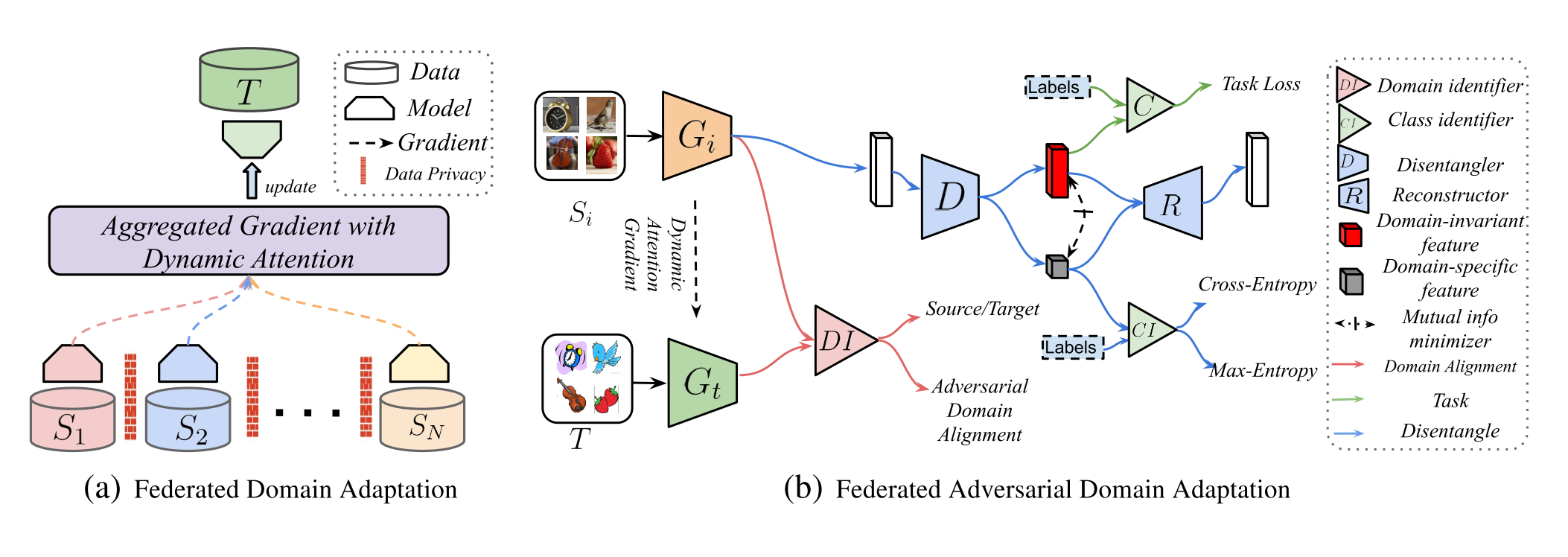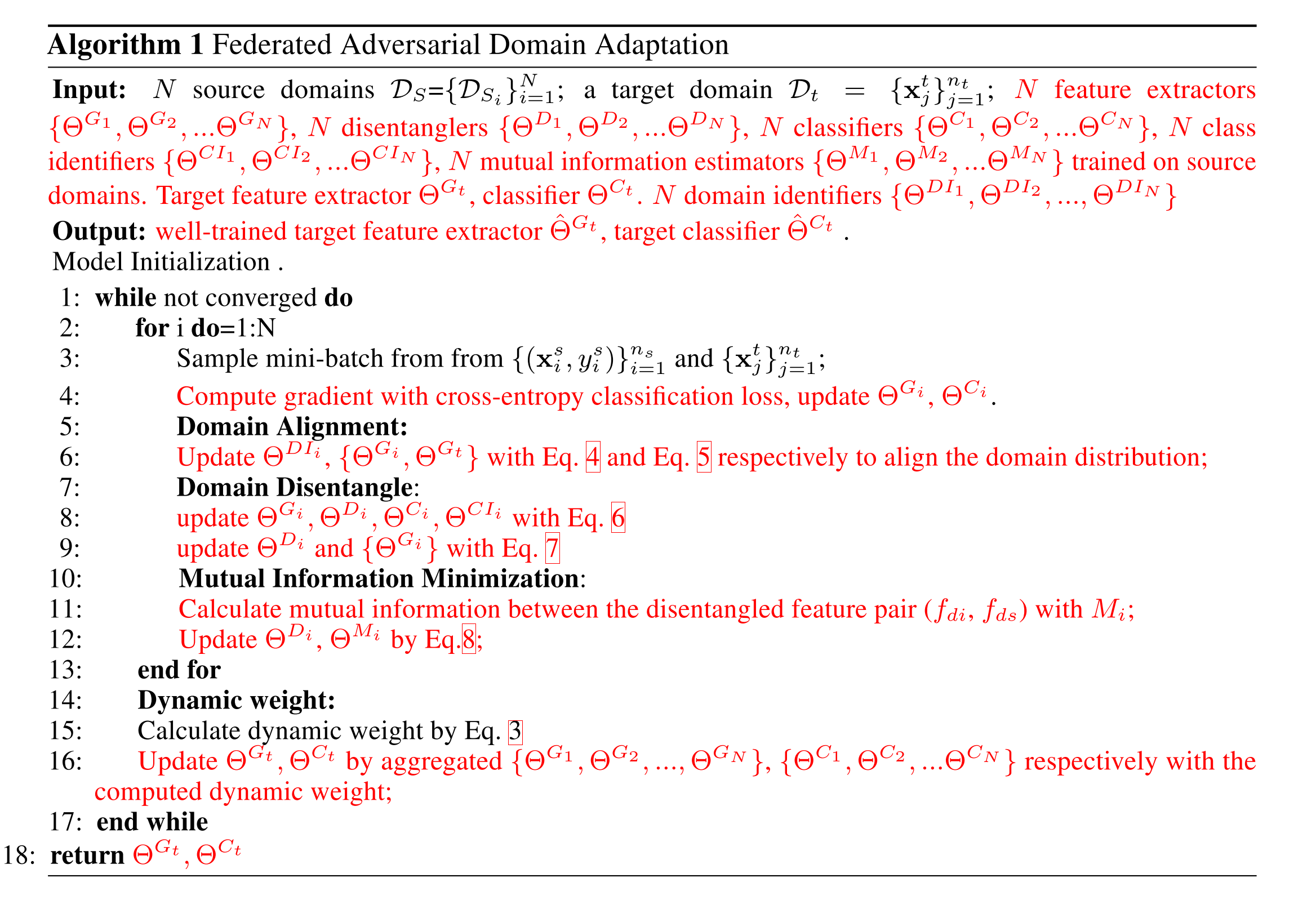• English：

# Federated Adversarial Domain Adaptation

## Introduction

1. 数据的存放是locally，而且无法分享。
2. 模型的参数是在不同节点上独立训练，并且以不同的速度进行收敛，对于global模型的贡献取决于这两者域的相关性。
3. 由于通过源节点学习出来的知识是高度集成的，难以解构，可能会引起negative transfer。

1. 首先从理论角度对联邦域适应问题进行分析，并且提供一个泛化的，通用的界限。
2. 收到理论效果的启发，提出一种有效的自适应算法，该算法基于对抗性适应和应用于联邦学习环境的表征解构技术。
3. 设计一个动态注意力模型来应对联邦学习系统中不断变化的收敛速率。png1

## Realated Work

Unsupervised Domain Adaptation: UDA的目的是将知识从已经标记的数据域迁移到一个未标记的数据域。

Federated Learning:不多介绍了

Feature Disentanglement:特征解构，神经网络通过复杂的隐藏层提取出来的特征，通常是高度集成的。因此通过学习解构的特征可以帮助一出一些不相关，或者是特定域的知识，然后对需要的域知识进行建模。

## Generalization Bound for FDA

1. 首先回顾一下以单数据源为背景的自适应问题的理论误差范围的定义
2. 然后描述在无监督的联邦域自适应情况下的误差范围的定义

## Federated Adversarial Domain Adaptation$I=\sum_{r=1}^{k} \frac{1}{2 n_{r}} \sum_{i, j \in C_{r}}\left\|f_{i}^{t}-f_{j}^{t}\right\|_{2}$

$I_{i}^{g a i n}=I_{i}^{p-1}-I_{i}^{p}$

$\text { Softmax }\left(I_{1}^{\text {gain }}, I_{2}^{\text {gain }}, \ldots, I_{N}^{\text {gain}}\right)$

$\underset{\Theta^{D I_{i}}{L}}{L_{a d v_{D I}}\left(\mathbf{X}^{S_{i}}, \mathbf{X}^{T}, G_{i}, G_{t}\right)=-\mathbb{E}_{\mathbf{x}^{s_{i} \sim \mathbf{X}^{s_{i}}}}\left[\log D I_{i}\left(G_{i}\left(\mathbf{x}^{s_{i}}\right)\right)\right]-\mathbb{E}_{\mathbf{x}^{t} \sim \mathbf{x}^{t}}\left[\log \left(1-D I_{i}\left(G_{t}\left(\mathbf{x}^{t}\right)\right)\right)\right]}$

$\underset{\Theta^{G}{G}_{i}, \Theta^{G_{t}}}{L}\left(\mathbf{X}^{S_{i}}, \mathbf{X}^{T}, D I_{i}\right)=-\mathbb{E}_{\mathbf{x}^{s_{i} \sim \mathbf{X}^{s_{i}}}\left[\log D I_{i}\left(G_{i}\left(\mathbf{x}^{s_{i}}\right)\right)\right]-\mathbb{E}_{\mathbf{x}^{t} \sim \mathbf{X}^{t}}\left[\log D I_{i}\left(G_{t}\left(\mathbf{x}^{t}\right)\right)\right]}$

$\begin{array}{c}{L_{cross entropy}} \\ {\Theta^{G_i}, \Theta^{D_i}, \Theta^{C_i}, \Theta^{CI_i}}\end{array}=-\mathbb{E}_{\left(\mathbf{x}^{s_i}, \mathbf{y}^{s_i}\right) \sim \widehat{\mathcal{D}}_{s_i}} \sum_{k=1}^{K} \mathbb{1}\left[k=\mathbf{y}^{s_{i}}\right] \log \left(C_{i}\left(f_{d i}\right)\right)-\mathbb{E}_{\left(\mathbf{x}^{s} i, \mathbf{y}^{s} i\right) \sim \widehat{\mathcal{D}}_{s_{i}}} \sum_{k=1}^{K} \mathbb{1}\left[k=\mathbf{y}^{s_{i}}\right] \log \left(C I_{i}\left(f_{d s}\right)\right)$

$\underset{\Theta^{D} i, \Theta^{G_{i}}}{L_{e n t}}=-\frac{1}{N_{s_{i}}} \sum_{j=1}^{N_{s_{i}}} \log C I_{i}\left(f_{d s}^{j}\right)=-\frac{1}{N_{s_{i}}} \sum_{j=1}^{N_{s_{i}}} \log C I_{i}\left(D_{i}\left(G_{i}\left(\mathbf{x}^{s_{i}}\right)\right)\right)$

$T_{\theta}: \widehat{I(\mathcal{P} ; \mathcal{Q})}_{n}=\sup _{\theta \in \Theta} \mathbb{E}_{\mathbb{P}_{\mathcal{P} Q}^{(n)}}\left[T_{\theta}\right]-\log \left(\mathbb{E}_{\mathbb{P}_{P}^{(n)} \otimes \mathbb{P}_{Q}^{(n)}}\left[e^{T_{\theta}}\right]\right)$

$I(\mathcal{P} ; \mathcal{Q})=\iint \mathbb{P}_{\mathcal{P} \mathcal{Q}}^{n}(p, q) T(p, q, \theta)-\log \left(\iint \mathbb{P}_{\mathcal{P}}^{n}(p) \mathbb{P}_{\mathcal{Q}}^{n}(q) e^{T(p, q, \theta)}\right)$

$I(\mathcal{P}, \mathcal{Q})=\frac{1}{n} \sum_{i=1}^{n} T(p, q, \theta)-\log \left(\frac{1}{n} \sum_{i=1}^{n} e^{T\left(p, q^{\prime}, \theta\right)}\right)$

To be continued

-------The end of this article  Thank you for your reading-------
• 本文作者： Eree
• 本文链接： https://ereebay.me/posts/62459/
• 版权声明： 本博客所有文章除特别声明外，均采用 BY-NC-SA 许可协议。转载请注明出处！